﻿ 特高压直流故障对新能源送端电网暂态过电压作用机理

# 特高压直流故障对新能源送端电网暂态过电压作用机理Mechanism of UHVDC Fault on Transient Overvoltage of New Energy Grid

Abstract: With the continuous increase in the scale of new energy access to the power grid, UHVDC transmission becomes an important way to absorb it. Meanwhile, UHVDC faults causing transient overvoltage in the power grid of new energy delivery directly threaten the safe operation of new energy, which has become an urgent problem to be solved at present. Therefore, based on the background of Qishao UHVDC power supply network, this paper analyzes the mechanism of UHVDC fault on the transient overvoltage of new energy power supply network, and puts forward the control strategy for the transient overvoltage. Firstly, the equivalent model of new energy through DC is established. Secondly, the mechanism of UHVDC fault on transient overvoltage of power grid is analyzed. Based on this, the control strategy of transient overvoltage of power grid of new energy is proposed. Finally, the effectiveness of the control strategy is verified by simulation of Qishao UHVDC power grid.

1. 引言

2. 新能源经直流外送等效模型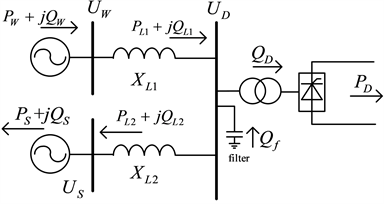Figure 1. Equivalent model of new energy DC outgoing terminal

$\left\{\begin{array}{l}{P}_{L1}={P}_{W}\\ {Q}_{L1}={Q}_{W}-{Q}_{l1}\end{array}$ (1)

${Q}_{l1}=\frac{{P}_{L1}^{2}+{Q}_{L1}^{2}}{{U}_{D}^{2}}{X}_{L1}$ (2)

$\left\{\begin{array}{l}{P}_{L2}={P}_{L1}-{P}_{D}\\ {Q}_{L2}={Q}_{W}-{Q}_{D}-\left({Q}_{l1}+{Q}_{l2}\right)+{Q}_{f}={Q}_{W}-\left({Q}_{l1}+{Q}_{l2}\right)\end{array}$ (3)

${Q}_{l2}=\frac{{P}_{L2}^{2}+{Q}_{L2}^{2}}{{U}_{S}^{2}}{X}_{L2}$ (4)

$\left\{\begin{array}{l}{P}_{L1}^{f}={P}_{L1}+\Delta {P}_{W}^{f}\\ {Q}_{L1}^{f}={Q}_{L1}+\Delta {Q}_{W}^{f}-\Delta {Q}_{l1}^{f}\\ {P}_{L2}^{f}={P}_{L2}+\Delta {P}_{W}^{f}-\Delta {P}_{D}^{f}\\ {Q}_{L2}^{f}={Q}_{L2}+\Delta {Q}_{W}^{f}+\left({Q}_{f}-\Delta {Q}_{D}^{f}\right)-\left(\Delta {Q}_{l1}^{f}+\Delta {Q}_{l2}^{f}\right)\end{array}$ (5)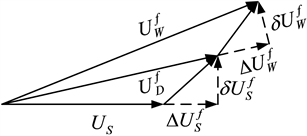Figure 2. Voltage vector diagram during failure

$\left\{\begin{array}{l}\Delta {U}_{S}^{f}=\frac{{Q}_{L2}^{f}{X}_{L2}}{{U}_{S}}\\ \delta {U}_{S}^{f}=\frac{{P}_{L2}^{f}{X}_{L2}}{{U}_{S}}\\ {U}_{D}^{f}=\sqrt{{\left({U}_{S}+\Delta {U}_{S}^{f}\right)}^{2}+{\left(\delta {U}_{S}^{f}\right)}^{2}}\end{array}$ (6)

$\left\{\begin{array}{l}\Delta {U}_{D}^{f}=\frac{{Q}_{L1}^{f}{X}_{L1}}{{U}_{D}^{f}}\\ \delta {U}_{D}^{f}=\frac{{P}_{L1}^{f}{X}_{L1}}{{U}_{D}^{f}}\\ {U}_{W}^{f}=\sqrt{{\left({U}_{D}^{f}+\Delta {U}_{D}^{f}\right)}^{2}+{\left(\delta {U}_{D}^{f}\right)}^{2}}\end{array}$ (7)

3. 特高压直流故障对送端电网暂态过电压的作用机理

3.1. 特高压直流换相失败/闭锁对送端电网暂态过电压作用机理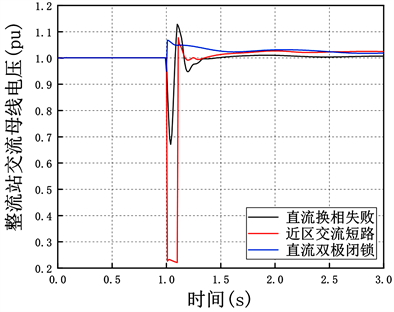Figure 3. AC bus voltage of rectifier station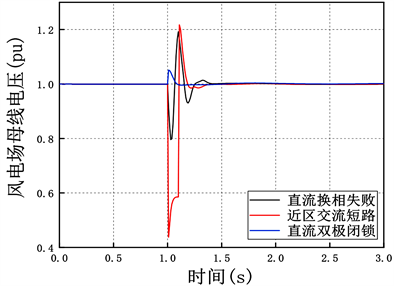Figure 4. AC bus voltage of wind farm

3.1.1. 直流换相失败

3.1.2. 直流闭锁

3.1.3. 整流站出口近区短路

3.2. 造成暂态过电压的主要因素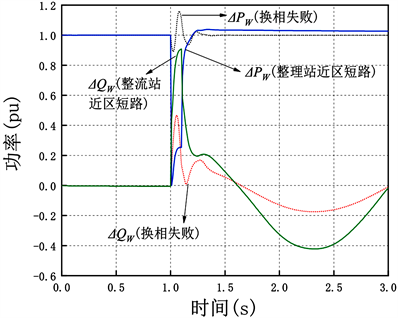Figure 5. Active and reactive power output of the doubly-fed fan at the feed end of the rectifier station

$\Delta {Q}_{W}=1.5\left(0.9-{U}_{W}^{f}\right){Q}_{WN}$ (8)

${Q}_{f}={\lambda }_{D}{P}_{D}$ (9)

4. 新能源直流送端电网暂态过电压控制策略

4.1. 整流站调相机控制策略

${Q}_{ref}={Q}_{f}-\underset{i=1}{\overset{N}{\sum }}{n}_{i}{Q}_{fli}$ (11)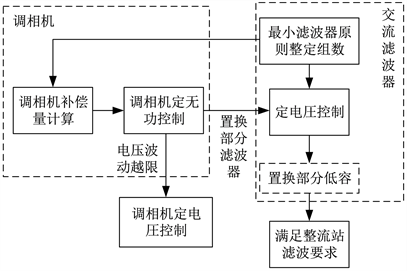Figure 6. Reactive power coordination control strategy with the camera in rectifier station

4.2. 风电场SVC控制策略

5. 实例仿真验证

5.1. 仿真背景Figure 7. Topological diagram of Qishao DC wind power plant

5.2. 暂态电压分布式控制策略仿真验证Figure 8. AC bus voltage of commutation failure rectifier station under different control strategies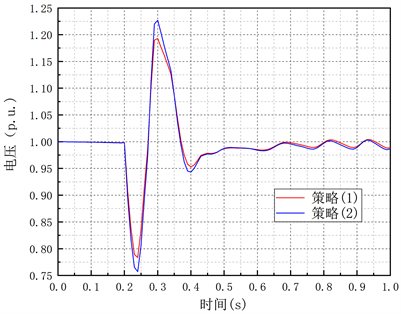Figure 9. Bus voltage of Qiaowan wind power plant due to failure of commutation with different control strategies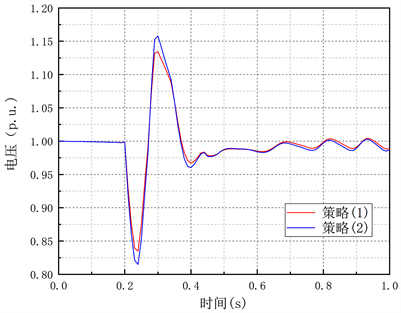Figure 10. Commutation failure of different control strategies dry busbar voltage in north wind power plant

6. 结论

1) 直流换相失败对直流送端电网近区风电场暂态压升的影响最为严重。

2) 直流换相失败时，送端暂态电压呈现先降低后升高的现象，期间风机低压穿越增加的无功出力与整流站滤波器盈余的大量无功是引起送端电网暂态过电压的主要因素。

3) 对整流站调相机和风电场SVC进行分布式控制可有效抑制直流故障引起的暂态过电压，仿真计算验证了控制策略的有效性。

 常海军, 霍超, 刘福锁, 柯贤波, 侯玉强, 牛拴保, 等. 提高弱送端电网暂态电压稳定水平的调相机优化配置研究[J]. 电力系统保护与控制. 2019, 47(6): 90-95.

 汤奕, 郑晨一, 楼伯良, 华文, 王龙飞. 抑制连续换相失败的直流功率控制策略[J]. 电网技术, 2019, 43(10): 3514-3522.

 屠竞哲, 张健, 刘明松, 易俊, 贺庆, 罗煦之, 等. 风火打捆直流外送系统直流故障引发风机脱网的问题研究[J]. 电网技术, 2015, 39(12): 3333-3338.

 索之闻, 刘建琴, 蒋维勇, 李志强, 杨林. 大规模新能源直流外送系统调相机配置研究[J]. 电力自动化设备, 2019, 39(9): 124-129.

 韩平平, 陈凌琦, 胡迪, 张炎. 直流闭锁暂态过电压对风电外送影响及其抑制措施[J]. 电力系统保护与控制, 2018, 46(5): 99-105.

 殷威扬, 杨志栋. 特高压直流工程无功平衡和补偿策略[J]. 高电压技术, 2006, 32(9): 50-54.

 张爱玲, 姚致清, 涂仁川, 关红兵. 云广特高压直流输电工程站控系统的设计缺陷及改进分析[J]. 电力系统保护与控制, 2011, 39(4): 117-123.

 Aamir, A., Qiao, L., Guo, C., Ur Rehman, A. and Yang, Z. (2019) Impact of Synchronous Condenser on the Dynamic Behavior of LCC-Based UHVDC System Hierarchically Connected to AC System. CSEE Journal of Power and Energy Systems, 5, 190-198.
https://doi.org/10.17775/CSEEJPES.2018.00420

 叶希, 鲁宗相, 乔颖, 李兢, 王丰, 罗伟. 大规模风电机组连锁脱网事故机理初探[J]. 电力系统自动化. 2012, 36(8): 11-17.

 何世恩, 董新洲. 大规模风电机组脱网原因分析及对策[J]. 电力系统保护与控制. 2012, 40(1): 131-137, 144.

 李丹, 贾琳, 许晓菲, 王蓓, 王宁, 谢旭. 风电机组脱网原因及对策分析[J]. 电力系统自动化. 2011, 35(22): 41-44.

 吴跨宇, 房乐, 卢岑岑, 沈轶君. 大型调相机励磁与无功电压协调控制策略探讨[J]. 浙江电力. 2018, 37(2): 36-41.

 Ma, Y., Ruan, L., Xiao, Y., Zhou, L., Wang, J. and Tao, Q. (2019) Modelling and Analysis of the UHVDC Transmission Receiving System Considering 300 Mvar Novel Synchronous Condenser. The Journal of Engineering, 2019, 955-960.
https://doi.org/10.1049/joe.2018.8866

 Wang, Q., Li, T., Tang, X., Liu, F. and Lei, J. (2019) Study on the Site Selection for Synchronous Condenser Responding to Commutation Failures of Multi-Infeed HVDC System. The Journal of Engineering, 2019, 1413-1418.
https://doi.org/10.1049/joe.2018.8807

 屠竞哲, 张健, 刘明松, 潘艳, 习工伟, 易俊. 考虑风机动态特性的大扰动暂态过电压机理分析[J]. 电力系统自动化, 2020, 44(11): 197-208.

Top